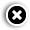# How to calculate the EMI on your loan

## Confused if your lender is charging you a fair amount as EMI? Money Today tells you how to calculate your instalment so that you can cross-check that with what you have been paying per month.Suraj Dutt, 29, a New Delhi-based store manager, bought a car in 2015 worth Rs 5.95 lakh. He made a down payment of Rs 1.5 lakh and took an auto loan for the rest of the amount at 12% interest per annum for four years. At present, he is paying an equated monthly instalment, or EMI, of Rs 11,700 per month. However, he has no way of knowing if the amount is correct or not.

Like Suraj, there are many people who are confused if their lender is charging them a fair amount as EMI. So, we decided to tell you how to calculate EMI so that you can cross-check that with what you have been paying per month. You can calculate your EMI by using a piece of software called Microsoft Excel or a mathematical formula.

USING EXCEL
One of the easiest ways of calculating the EMI is by using the Excel spreadsheet. In Excel, the function for calculating the EMI is PMT and not EMI. You need three variables. These are rate of interest (rate), number of periods (nper) and, lastly, the value of the loan or present value (pv).

The formula which you can use in excel is:

=PMT(rate,nper,pv).

Let us check the EMI of Suraj by using the above formula.

It must be noted that the rate used in the formula should be the monthly rate, that is, 12%/12=1% or 0.01.

The number of periods represents the number of EMIs.

=PMT(0.12/12, 4*12, 445,000)= 11,718

The result will come in negative or red, which indicates the cash outflow of the borrower.

Let's take another example. Suppose you are paying a quarterly instalment on a loan of Rs 10 lakh at 10% interest per annum for 20 years. In such a case, instead of 12, you should divide the rate by four and multiply the number of years by four. The equated quarterly instalment for the given figures will be =PMT(10%/4, 20*4, 10,00,000).

USING MATHEMATICAL FORMULA
Unfortunately, you cannot access the Excel spreadsheet everywhere. In such a case, you can use your mathematical mind or an electronic calculator to know how much the EMI comes to. The mathematical formula for calculating EMIs is:

EMI = [P x R x (1+R)^N]/[(1+R)^N-1], where P stands for the loan amount or principal, R is the interest rate per month [if the interest rate per annum is 11%, then the rate of interest will be 11/(12 x 100)], and N is the number of monthly instalments. When you use the above formula, you will get the same result that you will get in the Excel spreadsheet.

• Print
• COMMENT
##### How to get credit report errors corrected